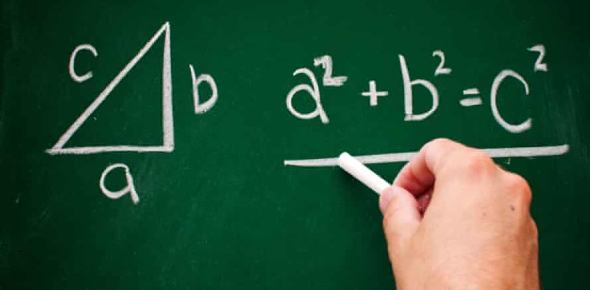Maths Quiz For Primary Class Students

26 Questions | Total Attempts: 1665SettingsMathematics is a fundamental part of our academic curriculum from the start of our school time. It is very important to know the basic level of maths as it helps our mental growth in early years, several reports suggest. This Maths Quiz is especially designed for primary class students to brush their mathematical skills, or prepare for an upcoming exam!

• 1.
The length and width of a class room is 15 meters and 10 meters respectively. Find the ratio between the two quantities.
• 2.
Give number of sides, corners and edges in an octagonal prism.
• 3.
Which techniques you will use to motivate the students?
• 4.
How can you make learning fun for the students?
• 5.
What will you do if the whole class is not getting the concept?
• 6.
Why should we consider you for the teaching job in our school?
• 7.
Which of the following values is NOT equal to 34(58+9)?
• A.

34 x 67

• B.

58(34+9)

• C.

34 x 58 + 34 x 9

• D.

2278

• 8.
If 3x=6x-15 then x=?
• A.

5

• B.

10

• C.

11

• D.

13

• 9.
The number of milliliters in 1 liter is:
• A.

10,000

• B.

1,000

• C.

0.1

• D.

0.01

• 10.
Which of the following would be an appropriate unit to measure sugar for a cookie recipe?
• A.

Liters

• B.

Cups

• C.

Quarts

• D.

Kilograms

• 11.
7-37 + -47 equals
• A.

64

• B.

-77

• C.

65

• D.

-75

• 12.
If Leah is 6 years older than Sue, and John is 5 years older than Leah, and the total of their ages is 41. Then how old is Sue?
• A.

8

• B.

10

• C.

14

• D.

19

• 13.
If r = 5 z then 15 z = 3 y, then r =
• A.

Y

• B.

2 y

• C.

5 y

• D.

10 y

• 14.
Which of the following is not a fraction equivalent to 3/4?
• A.

6/8

• B.

9/12

• C.

12/18

• D.

21/28

• 15.
Which of the following fractions is the equivalent of 0.5%?
• A.

1/20

• B.

1/200

• C.

1/2000

• D.

1/5

• 16.
You purchase a car making a down payment of \$3,000 and 6 monthly payments of \$225. How much have you paid so far for the car?
• A.

\$3225

• B.

\$4350

• C.

\$5375

• D.

\$6550

• 17.
What is the square root of 81?
• A.

3

• B.

7

• C.

9

• D.

21

• 18.
If a circle has the diameter of 8, what is the circumference?
• A.

6.28

• B.

12.56

• C.

25.13

• D.

50.24

• 19.
Round 907.457 to the nearest tens place
• A.

908.0

• B.

910

• C.

907.5

• D.

900

• 20.
Over the course of a week, Fred spent \$28.49 on lunch. What was the average cost per day?
• A.

\$4.07

• B.

\$3.57

• C.

\$6.51

• D.

\$2.93

• 21.
Find the missing term in the following sequence: 4, 9, 19, __, 79
• A.

36

• B.

37

• C.

38

• D.

39

• 22.
Of the following fractions, which is less than 2/3?
• A.

7/8

• B.

5/6

• C.

3/4

• D.

3/5

• 23.
Explanatory methods are used in?
• A.

Subject centered curriculum

• B.

Learner centered curriculum

• C.

Activity centered curriculum

• D.

None

• 24.
An angle greater than 180 degrees is known as
• A.

Acute angle

• B.

Obtuse angle

• C.

Reflex angle

• D.

None of these

Related TopicsBack to top